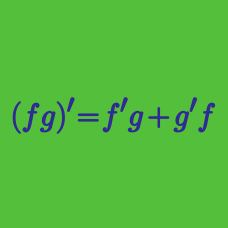Calculus

# Differentiation Rules Warmup

If $f(x) = 3x^2$, which of the following is equal to $f'(5)$?

If $f(x) = e^{x^2}$, which of the following is equal to $f'(1)$?

Hint. If $f(x) = e^{x},$ then $f'(x) = e^{x}.$

If $q(x) = \frac{x^2}{e^x}$, which of the following is equal to $q'(x)$?

Hint: If $g(x) = e^x$, then $g'(x) = e^x.$

If $h(x) = \color{#3D99F6}{(x^3 + x^2)}\color{#D61F06}{(5x + 1)}$, which of the following is equal to $h'(x)$?

$A. h'(x) = \color{#3D99F6}{(3x^2 + 2x)}\cdot \color{#D61F06}{(5)}.$

$B. h'(x) = \color{#3D99F6}{(x^3 + x^2)}\cdot \color{#D61F06}{(5x + 1)} + \color{#3D99F6}{(3x^2+2x)}\cdot \color{#D61F06}{(5)}.$

$C. h'(x) = \color{#3D99F6}{(3x^2 + 2x)}\cdot \color{#D61F06}{(5x + 1)} + \color{#3D99F6}{(x^3+x^2)}\cdot \color{#D61F06}{(5)}.$

$(f \square g)' = f' \square g'$

Which operators could replace the square to make a statement that is true for all differentiable functions $f$ and $g$?

×